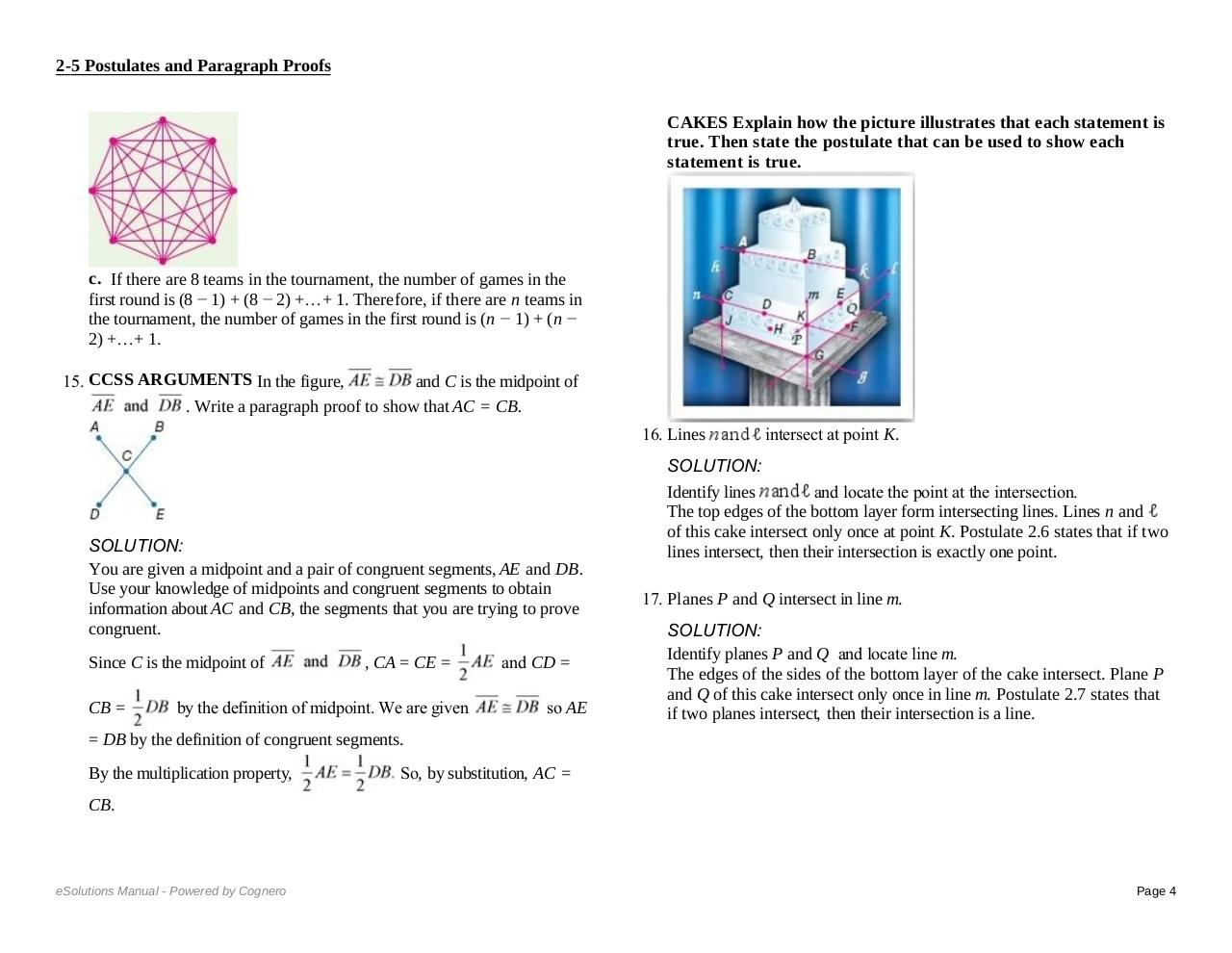# 2 5ALL.pdfPage 1 2 3 45618

#### Text preview

will play with the 6 other teams, as the game between the first and the
second team has already been counted. Similarly, the third team will play
with 5 other teams, and so on. So, the total number of games will be 7 + 6
+ 5 + 4 + 3and
+ 2 +Paragraph
1 = 28. So,Proofs
in the first round there will be 28 games.
2-5 Postulates
b. Postulate 2.1 states that through any two points, there is exactly one
line. Plot 8 points and draw lines joining any two points.

= DB by the definition of congruent segments.
By the multiplication property,

So, by substitution, AC =

CB.
CAKES Explain how the picture illustrates that each statement is
true. Then state the postulate that can be used to show each
statement is true.

c.  If there are 8 teams in the tournament, the number of games in the
first round is (8 − 1) + (8 − 2) +…+ 1. Therefore, if there are n teams in
the tournament, the number of games in the first round is (n − 1) + (n −
2) +…+ 1.
15. CCSS ARGUMENTS In the figure,
and C is the midpoint of
. Write a paragraph proof to show that AC = CB.
16. Lines

SOLUTION:
You are given a midpoint and a pair of congruent segments, AE and DB.
Use your knowledge of midpoints and congruent segments to obtain
information about AC and CB, the segments that you are trying to prove
congruent.
Since C is the midpoint of
CB =

, CA = CE =

by the definition of midpoint. We are given

and CD =
so AE

= DB by the definition of congruent segments.
By the multiplication property,

So, by substitution, AC =

CB.
CAKES Explain how the picture illustrates that each statement is
true. Then state the postulate that can be used to show each
statement is true.

intersect at point K.

SOLUTION:
Identify lines
and locate the point at the intersection.
The top edges of the bottom layer form intersecting lines. Lines n and
of this cake intersect only once at point K. Postulate 2.6 states that if two
lines intersect, then their intersection is exactly one point.
17. Planes P and Q intersect in line m.
SOLUTION:
Identify planes P and Q  and locate line m.
The edges of the sides of the bottom layer of the cake intersect. Plane P
and Q of this cake intersect only once in line m. Postulate 2.7 states that
if two planes intersect, then their intersection is a line.
18. Points D, K, and H determine a plane.
SOLUTION:
Locate points D, K, and H.
The bottom left part of the cake is a side. This side contains the points D,
K, and H and forms a plane. Postulate 2.2 states that through any three
noncollinear points, there is exactly one plane.
19. Point D is also on the line n through points C and K.
SOLUTION:

Page 4